# Proving that R(4,4)>17

Our task is to show that 17 points are not enough to guarantee a set of four friends or a set of four strangers. So we need to show that there is a graph of 17 vertices which contains neither.

Seventeen vertices is a lot, and even if we successfully drew such a graph, we would not be able to just look at it to verify it—there would simply be too many edges for us to make sense of.

Instead of drawing a graph, then, we can construct one by defining it in a clever way. Consider a graph of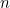vertices, which are labelled with the integers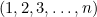, placed in a circle.### An easy example

We can now decide which vertices are connected by referring to their labels. For example, consider a graph of 7 vertices. Let’s connect two vertices with a blue edge if and only if they are "3" away from one another on our circle. Since we drew them in a circle, this means that 1 shares an edge with 4, 2 shares an edge with 5, etc. Since we have defined our circle such that vertex 7 appears right before vertex 1, we would also say that 7 shares an edge with 3. This is analogous to modular arithmetic. Here is what our graph looks like with the blue edges:All other edges should be coloured red. How can we define this without drawing it? Well, two vertices have a blue edge if they are 3 away from one another. That means they don’t have a blue edge if they are 2 away from one another—this edge must be red. Continuing in this way, we get that two vertices share a red edge if and only if they are 1 or 2 away from each other.Can we do the same for distances of 4, 5, 6, and 7? Well, every node is exactly 7 away from itself, and we aren’t connecting any vertices to themselves, so that one is out of the question. What about 4? Start at any vertex, and go 4 to the right. Now starting at that same vertex, go 3 to the left. You’ll find that you end up at the same destination. Any vertex that is 4 or more away from another vertex is actually a smaller distance away from that same vertex (going in the other direction). So a distance of 4 is equivalent to a distance of 3, in the way we defined this graph. More generally, a distance of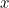is the same as a distance of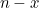.So this gives us a complete graph. Now how can we check for mutual friends or strangers? It would be cumbersome to try to look at the messy knot of edges and seek one out. Instead, we can again refer to how we defined the edges between vertices. Let's say we have 3 mutual strangers (a red triangle). That means that we have three vertices that all share a red edge with one another. Take one of these vertices and call it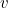.

We have defined our graph such that vertices only share a red edge if they differ by 1 or 2. That means the three vertices are of form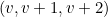,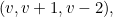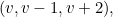or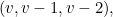sinceshares an edge with both of the other vertices. Let's consider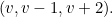Clearly,shares an edge with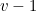and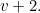It remains to show thatand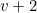share an edge. Again, as we defined the graph, they only share a red edge if they differ by 1 or 2. But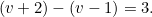They differ by 3, so they do not share a red edge. So this is not a red triangle.

Similarly we can take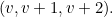In this case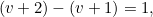so they do share an edge, and we have a red triangle. We have reduced the problem of checking for mutual friends or strangers to finding or not finding certain subsets of integers. In the image below, I have highlighted a triangle of this form found in the graph. Note that because of the symmetry in the way we defined the graph, this triangle exists starting at any vertex.### The proof

It's time to make the coup de grace and use this tool to find a graph of 17 vertices that does not contain four mutual friends or strangers. There is a special graph of the type we explored called a Paley graph, defined for graphs with a prime number of vertices (in particular, primes that are 1 greater than a multiple of 4), which specifies how to choose the connection rules.

List all the possible distances between vertices in our labelled circle graph: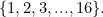Now square each of these and take the remainder when divided by 17 (denoted mod 17). For example,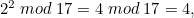and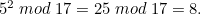Now we have a new set of distances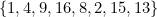Remember, a distanceis the same as the distancein these graphs (where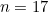), so we can write this list of distances as: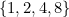This set defines our connections: we connect two vertices if they differ by one of these numbers, which is equivalent to saying they have a distance of 1, 2, 4, or 8. Let's say these are the red edges. Does this graph contain four mutual friends then? Suppose it does. The four mutual friends must be of the form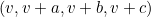where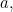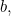andare distinct elements of the set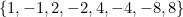. The differences between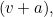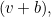and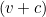must also all be elements of this list (since they supposedly share an edge). These differences are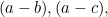andIf we can show that, no matter which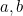andwe pick, one of these valuesor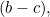is not in the list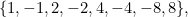then we know there is no set of our mutual friends. Well, if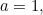either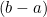or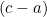is not in the list (for example, (4-1) = 3, which is not in the list. Think about this and it will become obvious). There may be an eloquent argument for why none of the remaining sets work, but here are all 20 possibilities, along with the difference that is not in the set, so it is clear what we are doing.Exhausting right? I will admit that this part is best left to a computer. Regardless, we've seen that no matter what three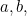andwe pick, there is some difference that is not in the list. So there can be no four mutual friends! Hoorah!

Must we again suffer this busywork to determine if there are four mutual strangers? The answer, fortunately, is NO! Paley graphs have the remarkable property that they are isomorphic to their complement. What that means is that the graph we draw with red edges will actually be the exact same one as the one with blue edges, just with the vertices moved around. Don't believe me? Think back to the example which showed that R(3,3) >5.Although you probably didn't know it at the time, this is precisely the Paley graph for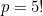If we label the vertices and list the possible distances,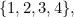and then square them mod 5, we get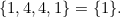So connect vertices if they are a distance of 1 from each other. That's precisely what we did, and it is quite evident in the red edges! The blue edges then, connect vertices that differ by 2 or 3, giving us this star in the middle. But look:This is actually the exact same graph as the one with the red edges, which we can see now that the vertices are rearranged! A rigorous proof that Paley graphs are self-complementary is a beautiful exercise, and I would strongly recommend it for anyone with a basic understanding of number and group theory. Back to our graph of 17 vertices, then, we know it contains no four mutual friends, and the graph defined by strangers is in fact the same graph (just colored red), so it contains no four mutual strangers! We have now shown that R(4, 4) > 17, which combined with previous results, proves that R(4,4) = 18.